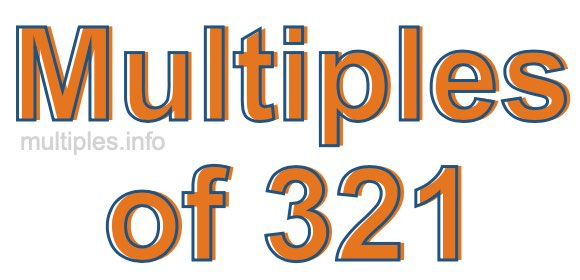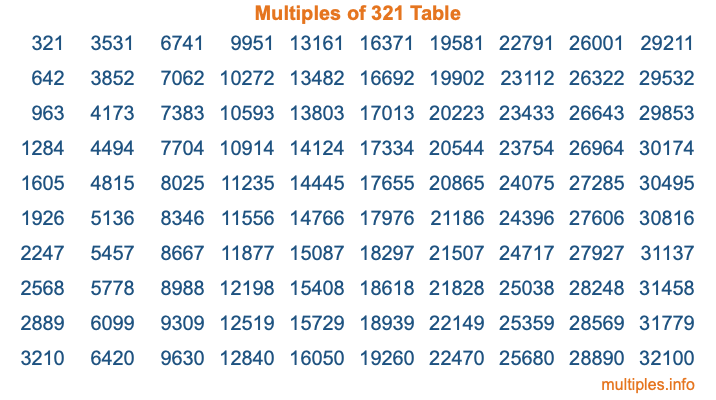Multiples of 321Welcome to the Multiples of 321 page. Here we will first teach you everything you will ever need to know about the multiples of 321, and then give you a study guide summary of everything we taught you to make sure you remember it all. Use this page to look up facts and learn information about the multiples of 321. This page will make you a multiples of three hundred twenty-one expert!

Definition of Multiples of 321
Multiples of 321 are all the numbers that when divided by 321 equal an integer. Each of the multiples of 321 are called a multiple. A multiple of 321 is created by multiplying 321 by an integer.

Therefore, to create a list of multiples of 321, you start with 1 multiplied by 321, then 2 multiplied by 321, then 3 multiplied by 321, and so on for as long as you want. Thus, the list of the first five multiples of 321 is 321, 642, 963, 1284, and 1605. To see a larger list of multiples of 321, see the printable image of Multiples of 321 further down on this page. We also have a category where you can choose any nth multiple of 321.

Multiples of 321 Checker
The Multiples of 321 Checker below checks to see if any number of your choice is a multiple of 321. In other words, it checks to see if there is any number (integer) that when multiplied by 321 will equal your number. To do that, we divide your number by 321. If the the quotient is an integer, then your number is a multiple of 321.

Is  a multiple of 321?

Least Common Multiple of 321 and ...
A Least Common Multiple (LCM) is the lowest multiple that two or more numbers have in common. This is also called the smallest common multiple or lowest common multiple and is useful to know when you are adding our subtracting fractions. Enter one or more numbers below (321 is already entered) to find the LCM.

Check out our LCM Calculator if you need more details about the Least Common Multiple or if you need the LCM for different numbers for adding and subtraction fractions.

nth Multiple of 321
As we stated above, 321 is the first multiple of 321, 642 is the second multiple of 321, 963 is the third multiple of 321, and so on. Enter a number below to find the nth multiple of 321.

th multiple of 321

Multiples of 321 vs Factors of 321
321 is a multiple of 321 and a factor of 321, but that is where the similarities end. All postive multiples of 321 are 321 or greater than 321. All positive factors of 321 are 321 or less than 321.

Below is the beginning list of multiples of 321 and the factors of 321 so you can compare:

Multiples of 321: 321, 642, 963, 1284, 1605, etc.

Factors of 321: 1, 3, 107, 321

As you can see, the multiples of 321 are all the numbers that you can divide by 321 to get a whole number. The factors of 321, on the other hand, are all the whole numbers that you can multiply by another whole number to get 321.

It's also interesting to note that if a number (x) is a factor of 321, then 321 will also be a multiple of that number (x).

Multiples of 321 vs Divisors of 321
The divisors of 321 are all the integers that 321 can be divided by evenly. Below is a list of the divisors of 321.

Divisors of 321: 1, 3, 107, 321

The interesting thing to note here is that if you take any multiple of 321 and divide it by a divisor of 321, you will see that the quotient is an integer.

Multiples of 321 Table
Below is an image of the first 100 multiples of 321 in a table. The table is in chronological order, column by column. The first column has the first ten multiples of 321, the second column has the next ten multiples of 321, and so on.The Multiples of 321 Table is also referred to as the 321 Times Table or Times Table of 321. You are welcome to print out our table for your studies.

Negative Multiples of 321
Although not often discussed or needed in math, it is worth mentioning that you can make a list of negative multiples of 321 by multiplying 321 by -1, then by -2, then by -3, and so on, to get the following list of negative multiples of 321:

-321, -642, -963, -1284, -1605, etc.

Multiples of 321 Summary
Below is a summary of important Multiples of 321 facts that we have discussed on this page. To retain the knowledge on this page, we recommend that you read through the summary and explain to yourself or a study partner why they hold true.

There are an infinite number of multiples of 321.

A multiple of 321 divided by 321 will equal a whole number.

321 divided by a factor of 321 equals a divisor of 321.

The nth multiple of 321 is n times 321.

The largest factor of 321 is equal to the first positive multiple of 321.

321 is a multiple of every factor of 321.

321 is a multiple of 321.

A multiple of 321 divided by a divisor of 321 equals an integer.

321 divided by a divisor of 321 equals a factor of 321.

Any integer times 321 will equal a multiple of 321.

Multiples of a Number
Here you can get the multiples of another number, all with the same attention to detail as we did for multiples of 321 on this page.

Multiples of
Multiples of 322
Did you find our page about multiples of three hundred twenty-one educational? Do you want more knowledge? Check out the multiples of the next number on our list!